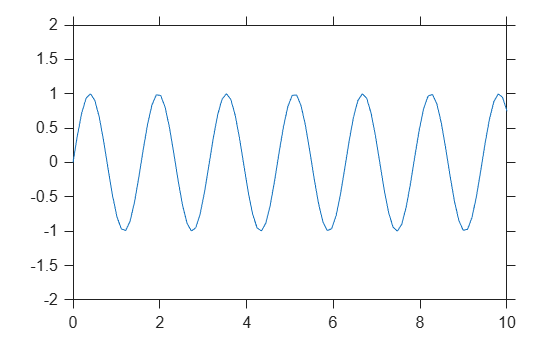# gca

Current axes or chart

## Syntax

``ax = gca``

## Description

example

````ax = gca` returns the current axes or chart for the current figure, which is typically the last one created or clicked with the mouse. Graphics functions, such as `title`, target the current axes or chart. Use `ax` to access and modify properties of the axes or chart. If axes or charts do not exist, then `gca` creates Cartesian axes.```

## Examples

collapse all

Plot a sine wave.

```x = linspace(0,10); y = sin(4*x); plot(x,y)```Set the font size, tick direction, tick length, and y-axis limits for the current axes. Use `gca` to refer to the current axes.

Note: Starting in R2014b, you can use dot notation to set properties. If you are using an earlier release, use the `set` function instead, such as `set(ax,'FontSize',12)`.

```ax = gca; % current axes ax.FontSize = 12; ax.TickDir = 'out'; ax.TickLength = [0.02 0.02]; ax.YLim = [-2 2];```## Output Arguments

collapse all

Current axes or chart, returned as an `Axes` object, a `PolarAxes` object, a `GeographicAxes` object, or a graphics object whose parent is a `Figure`, `Tab`, or `Panel` object, instead of an `Axes` object.

For example, a `HeatmapChart` object can be the current chart since the parent is typically a `Figure` object. A `Stem` object cannot be the current chart since the parent is an `Axes` object.

## Tips

• User interaction can change the current axes or chart. It is better to assign the axes or chart to a variable when you create it instead of relying on `gca`.

• Changing the current figure also changes the current axes or chart.

• Set axes properties after plotting since some plotting functions reset axes properties.

• To access the current axes or chart without forcing the creation of Cartesian axes, query the figure `CurrentAxes` property. MATLAB® returns an empty array if there is no current axes.

```fig = gcf; ax = fig.CurrentAxes;```
Starting in R2014b, you can query properties using dot notation. If you are using an earlier release, use the `get` function instead, such as `ax = get(fig,'CurrentAxes')`.